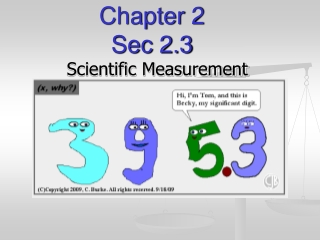DownloadDownload PresentationChapter 2 Sec 2.3

# Chapter 2 Sec 2.3

Download Presentation## Chapter 2 Sec 2.3

- - - - - - - - - - - - - - - - - - - - - - - - - - - E N D - - - - - - - - - - - - - - - - - - - - - - - - - - -
##### Presentation Transcript

1. Chapter 2 Sec 2.3 Scientific Measurement

2. 14. accuracy 15. precision 16. percent error 17. significant figures 18. scientific notation 19. directly proportional 20. inversely proportional Vocabulary

3. Section3 Using Scientific Measurements Chapter 2 Objectives • Distinguish between accuracy and precision. • Determine the number of significant figures in measurements. • Perform mathematical operations involving significant figures. • Convert measurements into scientific notation. • Distinguish between inversely and directly proportional relationships.

4. 2.3 Measurements and Their Uncertainty • A measurement is a quantity that has both a number and a unit • Measurements are fundamental to the experimental sciences. For that reason, it is important to be able to make measurements and to decide whether a measurement is correct. • International System of Measurement (SI) typically used in the sciences

5. Accuracy and Precision • Accuracy is the closeness of a measurement to thecorrect (accepted) value of quantity measured • Precision is a measure of how close a set of measurements are to one another • To evaluate the accuracy of a measurement, the measured value must be compared to the correct value. • To evaluate the precision of a measurement, you must compare the values of two or more repeated measurements

6. Accuracy and Precision

7. Accuracy and Precision Which target shows: 1. an accurate but imprecise set of measurements? 2. a set of measurements that is both precise and accurate? 3. a precise but inaccurate set of measurements? 4. a set of measurements that is neither precise nor accurate?

8. Where do these measurements come from? Recording Measurements

9. Section3 Using Scientific Measurements Chapter 2 Accuracy and Precision, continued Error in Measurement • Some error or uncertainty always exists in any measurement. • skill of the measurer • conditions of measurement • measuring instruments

10. F. Making Good Measurements • We can do 2 things: 1. Repeat measurement many times - reliable measurements get the same number over and over - this is PRECISION 2. Test our measurement against a “standard”, or accepted value - measurement close to accepted value is ACCURACY

11. Section3 Using Scientific Measurements Chapter 2 Accuracy and Precision, continued Percentage Error • Percentage error is calculated by subtracting the accepted value from the experimental value, dividing the difference by the accepted value, and then multiplying by 100.

12. G. Determining Error 1. Error = experimental value – accepted value *experiment value is measured in lab (what you got during experiment) * accepted value is correct value based on references (what you should have gotten) 2. Percent error = [Value experimental – Valueaccepted] x 100% Valueaccepted * Can put formula on notecard

13. Chapter 2 Section2 Units of Measurement 2.3 Practice Problems- Accuracy and Precision • (p.43) • 1. What is the percentage error for a mass measurement of 17.7 g, given that the correct value is 21.2 g?

14. Chapter 2 Section2 Units of Measurement 2.3 Practice Problems- Accuracy and Precision • (p.43) • 2. A volume is measured experimentally as 4.26 mL. What is the percentage error, given that the correct value is 4.15 mL?

15. Chapter 2 Section2 Units of Measurement 2.3 Practice Problems- Accuracy and Precision • (handout) • 3. Bruce’s three measurements are 19 cm, 20 cm, and 22 cm. Calculate the average value of his measurements and express the answer with the correct number of significant figures. • 4. Pete’s three measurements are 20.9 cm, 21.0 cm, and 21.0 cm. Calculate the average value of his measurements and express the answer with the correct number of significant figures. • Multiply the answer to problem #3 by the answer to problem #4. Express the answer in scientific notation with the correct number of significant figures. • Whose measurements are more precise? • The actual length of the object is 20 cm. Whose measurements are more accurate? • What is the error of Pete’s average measurement? • What is the percentage error of Pete’s average measurement? • Four boards each measuring 1.5 m are laid end to end. Multiply to determine the combined length of the boards, expressed with the correct number of significant figures.